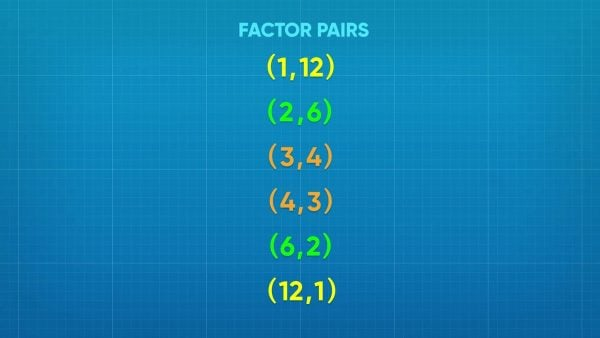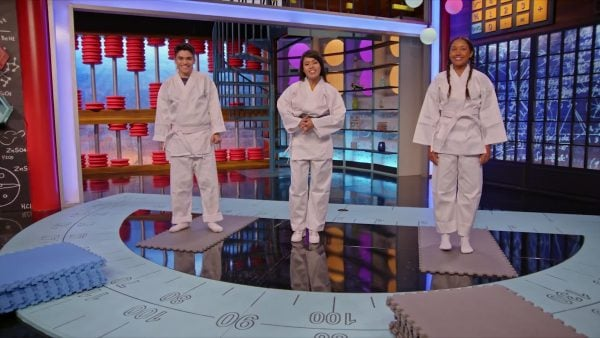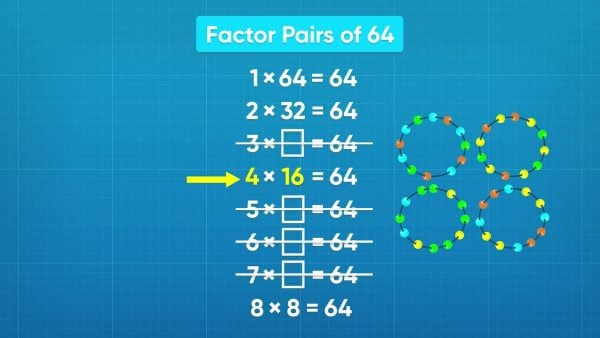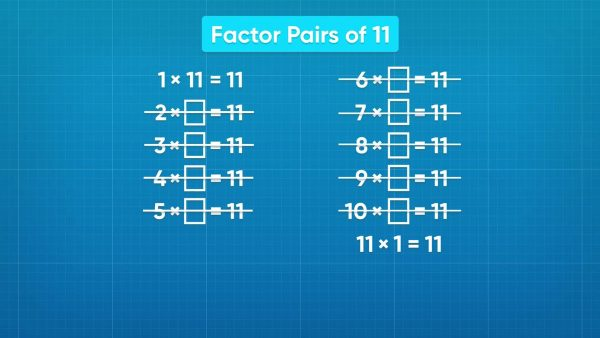Read about Finding Factor Pairs Within 100 | for kids Grade 3, 4, & 5
1%
It was processed successfully!WHAT ARE FACTOR PAIRS WITHIN 100?

Students learn to find all factor pairs for a whole number in the range 1-100. Students will also recognize that a whole number is a multiple of each of its factors. They will determine whether a given whole number in the range 1-100 is a multiple of a given one-digit number and whether a given whole number in the range 1-100 is prime or composite.

To better understand factor pairs within 100…

WHAT ARE FACTOR PAIRS WITHIN 100?. Students learn to find all factor pairs for a whole number in the range 1-100. Students will also recognize that a whole number is a multiple of each of its factors. They will determine whether a given whole number in the range 1-100 is a multiple of a given one-digit number and whether a given whole number in the range 1-100 is prime or composite. To better understand factor pairs within 100…

## LET’S BREAK IT DOWN!

### Relay TeamsLet's say that you have twelve people and you would like to make teams to compete in a relay race. What are all the different ways you can put twelve people into equal-sized groups? One way to solve this problem is to think of all the different factors of 12. Starting with 1, since 1 × 12 = 12, we know that we can make one team with twelve players. We next consider 2, and since 2 × 6 = 12, we can have two teams of 6. Since 3 × 4 = 12, we can make 3 teams with 4 people in each, and 4 teams with 3 players each, since 4 × 3 = 12. Five does not divide evenly into 12, but 6 × 2 = 12, so we can make 6 teams with 2 players each. We continue testing the next whole numbers, and find that 7, 8, 9, 10, and 11 don't divide evenly into 12. Finally, we can have 12 teams each with 1 player, since 12 × 1 = 12. These are all of the different ways to make teams from 12 people, and also all of the factor pairs of 12. Try this one yourself: How many different ways can you put 16 books into piles, where each pile has the same number of books.

Relay Teams Let's say that you have twelve people and you would like to make teams to compete in a relay race. What are all the different ways you can put twelve people into equal-sized groups? One way to solve this problem is to think of all the different factors of 12. Starting with 1, since 1 × 12 = 12, we know that we can make one team with twelve players. We next consider 2, and since 2 × 6 = 12, we can have two teams of 6. Since 3 × 4 = 12, we can make 3 teams with 4 people in each, and 4 teams with 3 players each, since 4 × 3 = 12. Five does not divide evenly into 12, but 6 × 2 = 12, so we can make 6 teams with 2 players each. We continue testing the next whole numbers, and find that 7, 8, 9, 10, and 11 don't divide evenly into 12. Finally, we can have 12 teams each with 1 player, since 12 × 1 = 12. These are all of the different ways to make teams from 12 people, and also all of the factor pairs of 12. Try this one yourself: How many different ways can you put 16 books into piles, where each pile has the same number of books.

### Karate MatsLet's say that you have 30 karate mats that need to be arranged into a rectangle. These rectangles can help us to find the factor pairs for the number 30. We need to find all the ways to make rectangles with the mats with the same number of mats in each row. Starting with 1, we know that we can make 1 row with 30 mats, since 1 × 30 = 30. This means that 1 and 30 are a factor pair of 30. If we make 2 rows, each row will have 15 mats, since 2 × 15 = 30. 2 and 15 are another factor pair. We can have 3 rows of 10 mats since 3 × 10 = 30, making 3 and 10 a factor pair. Four does not divide evenly into 30, but we can have 5 rows of 6 mats, since 5 × 6 = 30. We can also have a rectangle with 6 rows and 5 mats in each row, since 6 × 5 = 30. We notice that the 6 × 5 rectangle looks closest to a square, and closest to the 5 × 6 rectangle. This is when we know that we have found all the factor pairs, since the rectangle dimensions are reversing. Repeat the pattern to find the rest of the factor pairs. Try this one yourself: How many different large rectangles can be made from 12 smaller rectangles?

Karate Mats Let's say that you have 30 karate mats that need to be arranged into a rectangle. These rectangles can help us to find the factor pairs for the number 30. We need to find all the ways to make rectangles with the mats with the same number of mats in each row. Starting with 1, we know that we can make 1 row with 30 mats, since 1 × 30 = 30. This means that 1 and 30 are a factor pair of 30. If we make 2 rows, each row will have 15 mats, since 2 × 15 = 30. 2 and 15 are another factor pair. We can have 3 rows of 10 mats since 3 × 10 = 30, making 3 and 10 a factor pair. Four does not divide evenly into 30, but we can have 5 rows of 6 mats, since 5 × 6 = 30. We can also have a rectangle with 6 rows and 5 mats in each row, since 6 × 5 = 30. We notice that the 6 × 5 rectangle looks closest to a square, and closest to the 5 × 6 rectangle. This is when we know that we have found all the factor pairs, since the rectangle dimensions are reversing. Repeat the pattern to find the rest of the factor pairs. Try this one yourself: How many different large rectangles can be made from 12 smaller rectangles?Let's say you have 64 beads and you would like to split them up into equal groups to make bracelets. Finding the factor pairs for the number 64 will help us solve this problem. Starting with 1, we can make 1 bracelet with 64 beads (1 × 64 = 64), 2 bracelets with 32 beads each (2 × 32 = 64), 4 bracelets with 16 beads (4 × 16 = 64), and 8 bracelets with 8 beads (8 × 8 = 64). The other numbers between 1 and 8 do not divide evenly into 64. Once we see the same factor repeating in a factor pair—like we did with 8 × 8—we know that we have found all of the factor pairs. The factors begin to repeat after that, so we can stop. The factor pairs of 64 are 1 and 64, 2 and 32, 4 and 16, and 8 and 8. Try this one yourself: How many different ways can you divide 36 coins into bags with the same number of coins in each bag?

Beaded Bracelets Let's say you have 64 beads and you would like to split them up into equal groups to make bracelets. Finding the factor pairs for the number 64 will help us solve this problem. Starting with 1, we can make 1 bracelet with 64 beads (1 × 64 = 64), 2 bracelets with 32 beads each (2 × 32 = 64), 4 bracelets with 16 beads (4 × 16 = 64), and 8 bracelets with 8 beads (8 × 8 = 64). The other numbers between 1 and 8 do not divide evenly into 64. Once we see the same factor repeating in a factor pair—like we did with 8 × 8—we know that we have found all of the factor pairs. The factors begin to repeat after that, so we can stop. The factor pairs of 64 are 1 and 64, 2 and 32, 4 and 16, and 8 and 8. Try this one yourself: How many different ways can you divide 36 coins into bags with the same number of coins in each bag?

### Prime CupcakesLet's say that you want to use factor pairs to see how you can split 11 cupcakes into packs of the same number of cupcakes. We can use factor pairs to find out the different ways 11 can be divided into equal groups. Start at 1. We can have 1 pack of 11 cupcakes because 1 × 11 = 11. Try the other numbers: 2, 3, 4, 5, 6, 7, 8, 9, and 10. None of these number are factors of 11. None of them divide evenly into 11. We could have 11 packs with 1 cupcake each, since 11 × 1 = 11. That means 1 and 11 are the only factor pairs of 11. 11 is a prime number. A prime number is defined as a number whose only factors are 1 and itself. Try this one yourself: How many different teams, each with the same number of players, can you make from a class of 19 students?

Prime Cupcakes Let's say that you want to use factor pairs to see how you can split 11 cupcakes into packs of the same number of cupcakes. We can use factor pairs to find out the different ways 11 can be divided into equal groups. Start at 1. We can have 1 pack of 11 cupcakes because 1 × 11 = 11. Try the other numbers: 2, 3, 4, 5, 6, 7, 8, 9, and 10. None of these number are factors of 11. None of them divide evenly into 11. We could have 11 packs with 1 cupcake each, since 11 × 1 = 11. That means 1 and 11 are the only factor pairs of 11. 11 is a prime number. A prime number is defined as a number whose only factors are 1 and itself. Try this one yourself: How many different teams, each with the same number of players, can you make from a class of 19 students?

## FIND FACTOR PAIRS WITHIN 100 VOCABULARY

Factor
A whole number that is multiplied to get a product.
Factor Pairs
Two numbers that when multiplied result in a particular product.
Product
The result you get when you multiply two numbers together.
Multiples
The result of multiplying a whole number by different whole numbers.
Prime number
A number with exactly two different factors, 1 and the number itself.
Composite Number
A number that has more than two factors.

## FIND FACTOR PAIRS WITHIN 100 DISCUSSION QUESTIONS

### What is the difference between prime and composite numbers?

A prime number has only two factors, 1 and itself, while a composite number has more than 2 factors.

### When listing the factor pairs for a number, how do you know you have found all factor pairs?

The list reverses or starts to repeat with the factors in reverse order.

### When listing the factor pairs for a number using rectangles, how do you know you have found all factor pairs?

The rows and number of tiles in a row (dimensions of the rectangle) reverse order. You found the rectangles that are most square-like.

1, 3, 13, 39.

### List all the factor pairs of the number 64.

1 and 64, 2 and 32, 4 and 16, 8 and 8.
X

## Success

We’ve sent you an email with instructions how to reset your password.
Ok